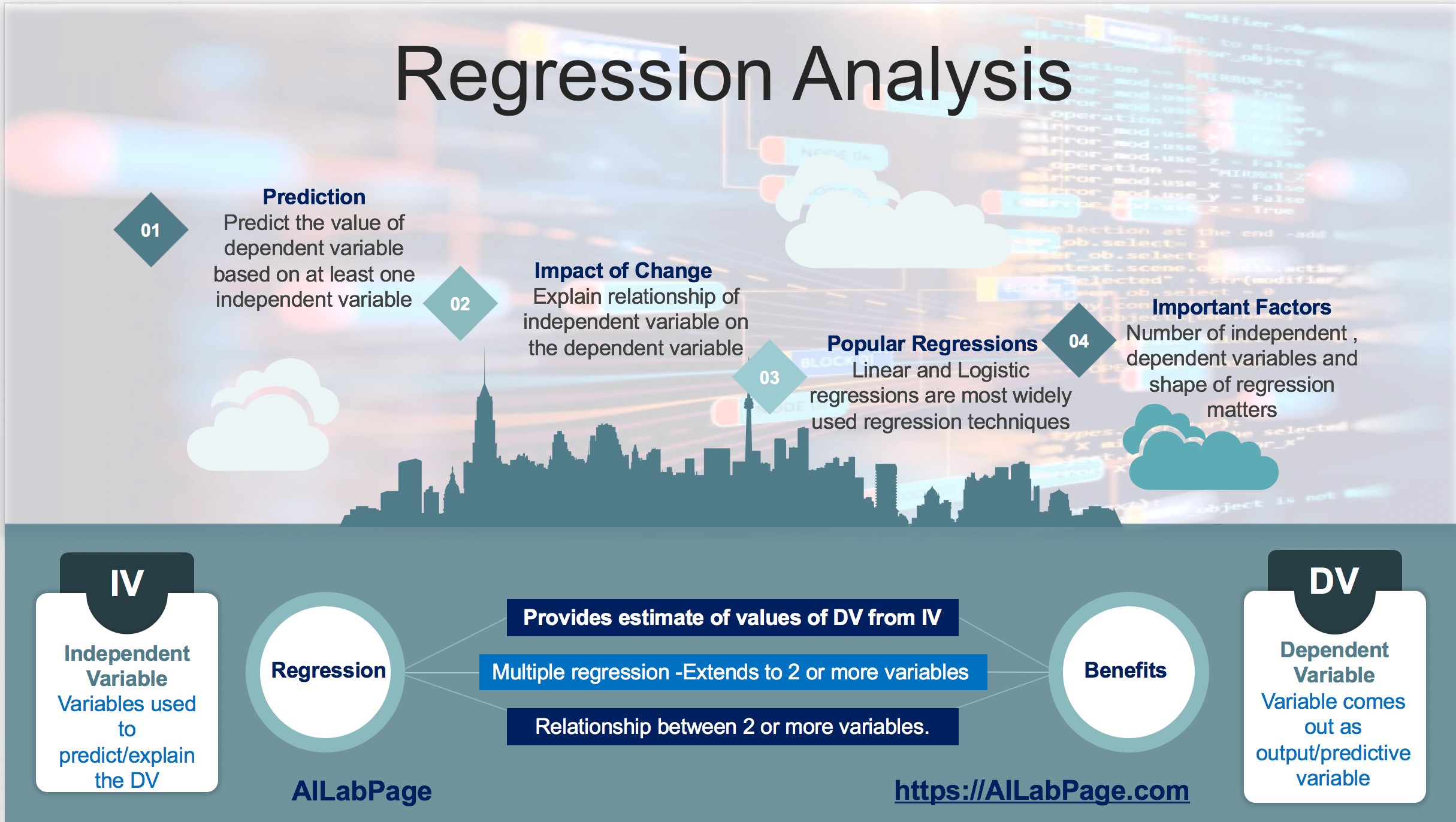# Tag: Regression### Machine Learning – Introduction to Regression Analysis

In Machine Learning regression analysis is used just to understand how to establish a relationship between independent variables and dependent variables. How independent variables going to effect dependent variables. Whether there is a strong relationship or just a casual relationship between IV and DV. Machine learning is a goal not a technique in this regard while linear regression is. ML can be achieved through many different means and techniques. In short, we can say this### Classification and Regression Demystified in Machine Learning

Classification predictive output is a label and for regression its a quantity. Generative algorithms can also be used as classifiers. It just so happens that they can do more than categorising the input data. Can call classification as sorting and regression as connecting technique as well.